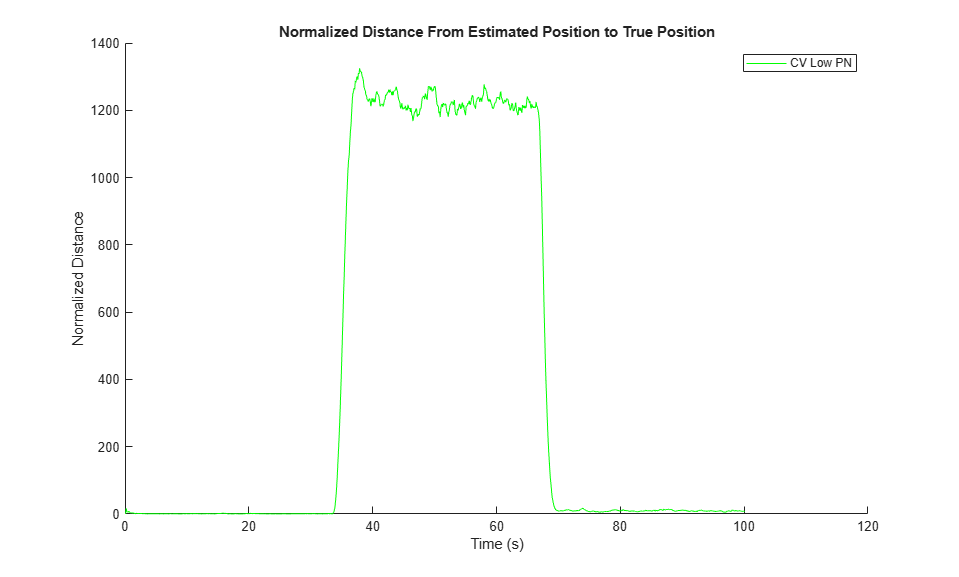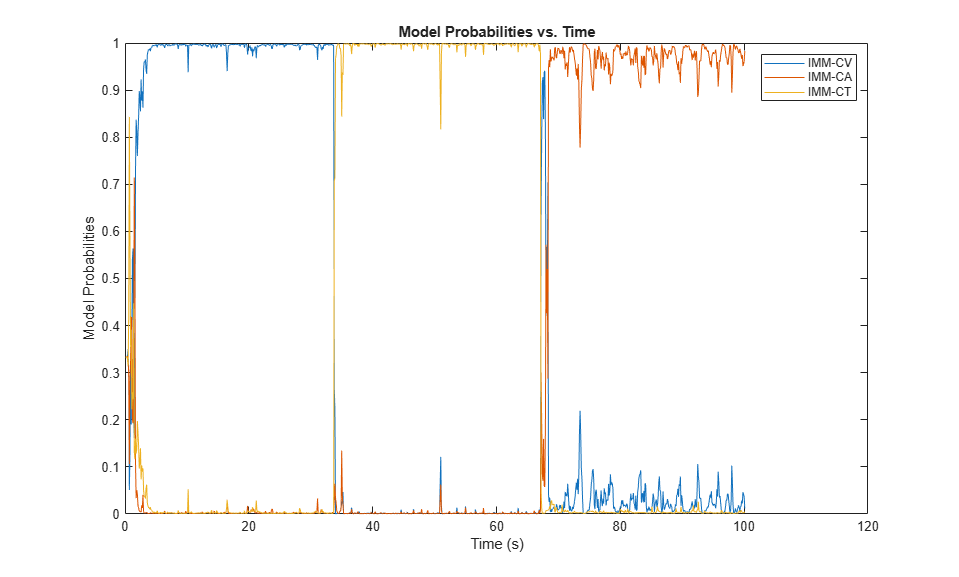Documentation

## Tracking Maneuvering Targets

This example shows how to track maneuvering targets using various tracking filters. The example shows the difference between filters that use a single motion model and multiple motion models.

### Define a Scenario

In this example, you define a single target that initially travels at a constant velocity of 200 m/s for 33 seconds, then enters a constant turn of 10 deg/s. The turn lasts for 33 seconds, then the target accelerates in a straight line at 3 m/s^2.

```[trueState, time, fig1] = helperGenerateTruthData; dt = diff(time(1:2)); numSteps = numel(time); figure(fig1) ```Define the measurements to be the position and add normal random noise with a standard deviation of 1 to the measurements.

```% Set the RNG seed for repeatable results s = rng; rng(2018); positionSelector = [1 0 0 0 0 0;0 0 1 0 0 0;0 0 0 0 1 0]; % Position from state truePos = positionSelector * trueState; measNoise = randn(size(truePos)); measPos = truePos + measNoise; ```

### Track Using a Constant-Velocity Filter

You define a `trackingEKF` with a constant-velocity motion model. You use the first measurement to define the initial state and state covariance, and set the process noise to be non-additive, to define the process noise in terms of the unknown acceleration in the x, y, and z components. This definition is similar to how the function `initcvekf` works.

```initialState = positionSelector' * measPos(:,1); initialCovariance = diag([1,1e4,1,1e4,1,1e4]); % Velocity is not measured cvekf = trackingEKF(@constvel, @cvmeas, initialState, ... 'StateTransitionJacobianFcn', @constveljac, ... 'MeasurementJacobianFcn', @cvmeasjac, ... 'StateCovariance', initialCovariance, ... 'HasAdditiveProcessNoise', false, ... 'ProcessNoise', eye(3)); ```

For each measurement, you predict the filter, calculate the distance of the predicted state from the true position, and correct the filter using the measurement to obtain the filtered estimate of the position.

```dist = zeros(1,numSteps); estPos = zeros(3,numSteps); for i = 2:size(measPos,2) predict(cvekf, dt); dist(i) = distance(cvekf,truePos(:,i)); % Distance from true position estPos(:,i) = positionSelector * correct(cvekf, measPos(:,i)); end figure(fig1); plot(estPos(1,:),estPos(2,:),'.g','DisplayName','CV Low PN') title('True and Estimated Positions') axis([5000 8000 -500 2500]) ```As depicted in the figure, the filter is able to track the constant velocity part of the motion very well, but when the target executes the turn, the filter estimated position diverges from the true position. You can see the distance of the estimate from the truth in the following plot. During the turn, at 33-66 seconds, the normalized distance jumps to very high values, which means that the filter is unable to track the maneuvering target.

```fig2 = figure; hold on plot((1:numSteps)*dt,dist,'g','DisplayName', 'CV Low PN') title('Normalized Distance From Estimated Position to True Position') xlabel('Time (s)') ylabel('Normalized Distance') legend ```### Increase the Process Noise

One possible solution is to increase the process noise. The process noise represents the unmodeled terms in the motion model. For a constant velocity model, these are the unknown acceleration terms. By increasing the process noise, you allow for larger uncertainty in the motion model, which causes the filter to rely on the measurements more than on the model. The following lines create a constant velocity filter, with high process noise values that correspond to about 5-G turn.

```cvekf2 = trackingEKF(@constvel, @cvmeas, initialState, ... 'StateTransitionJacobianFcn', @constveljac, ... 'MeasurementJacobianFcn', @cvmeasjac, ... 'StateCovariance', initialCovariance, ... 'HasAdditiveProcessNoise', false, ... 'ProcessNoise', diag([50,50,1])); % Large uncertainty in the horizontal acceleration dist = zeros(1,numSteps); estPos = zeros(3,numSteps); for i = 2:size(measPos,2) predict(cvekf2, dt); dist(i) = distance(cvekf2,truePos(:,i)); % Distance from true position estPos(:,i) = positionSelector * correct(cvekf2, measPos(:,i)); end figure(fig1) plot(estPos(1,:),estPos(2,:),'.c','DisplayName','CV High PN') axis([5000 8000 -500 2500]) ```Increasing the process noise significantly improves the filter's ability to track the target during the turn. However, there is a cost: the filter is less capable of smoothing out the measurement noise during the constant velocity period of the motion. Even though the normalized distance during the turn was significantly reduced, the normalized distance increased in the first 33 seconds, during the constant velocity period of the motion.

```figure(fig2) plot((1:numSteps)*dt,dist,'c','DisplayName', 'CV High PN') axis([0 100 0 50]) ```### Use an Interacting Motion-Model Filter

Another solution is to use a filter that can consider all motion models at the same time, called an interacting multiple-model (IMM) filter. The IMM filter can maintain as many motion models as you want, but typically is used with 2-5 motion models. For this example, three models are sufficient: a constant velocity model, a constant turn model, and a constant acceleration model.

```imm = trackingIMM('TransitionProbabilities', 0.99); % The default IMM has all three models % Initialize the state and state covariance in terms of the first model initialize(imm, initialState, initialCovariance); ```

You use the IMM filter in the same way that the EKF was used.

```dist = zeros(1,numSteps); estPos = zeros(3,numSteps); modelProbs = zeros(3,numSteps); modelProbs(:,1) = imm.ModelProbabilities; for i = 2:size(measPos,2) predict(imm, dt); dist(i) = distance(imm,truePos(:,i)); % Distance from true position estPos(:,i) = positionSelector * correct(imm, measPos(:,i)); modelProbs(:,i) = imm.ModelProbabilities; end figure(fig1) plot(estPos(1,:),estPos(2,:),'.m','DisplayName','IMM') ```The `trackingIMM` filter is able to track the maneuvering target in all three parts of the motion.

Examining the distance between the filter's predicted state and the true position, you see that the IMM filter is able to reduce the distance for all portions of the motion. In fact, the IMM filter is better at tracking the motion than both other constant velocity models used earlier.

```figure(fig2) hold on plot((1:numSteps)*dt,dist,'m','DisplayName', 'IMM') axis([0 100 0 50]) ```To better understand how the IMM filter works, plot the model probabilities as a function of time. The figure shows that filter is initialized with the three models having the same probability. As the filter is updated, it quickly converges to a very high probability that the model is a constant velocity model. After 33 seconds of motion, the constant velocity model is no longer true, and the probability of the constant turn model becomes very high for the duration of the turn. In the last section of the motion, during the constant acceleration maneuver, the IMM filter assigns a high probability that the motion is constant acceleration, but the filter is less certain about the correct motion model, and there is about 0.3 probability of a constant velocity motion.

```figure plot((1:numSteps)*dt, modelProbs) title('Model Probabilities vs. Time') xlabel('Time (s)') ylabel('Model Probabilities') legend('IMM-CV','IMM-CA','IMM-CT') % Return the RNG to its previous state rng(s) ```### Summary

This example showed you how to track a target maneuvering with constant turn and constant acceleration motion. The example showed how you can increase the process noise to capture the unknown maneuver with a constant velocity model. You also saw how to improve the tracking of a maneuvering target by using an IMM filter.

### Supporting Functions

`helperGenerateTruthData`

This function generates the ground truth trajectory.

```function [Xgt, tt, figs] = helperGenerateTruthData % Generate ground truth vx = 200; % m/s omega = 10; % deg/s acc = 3; % m/s/s dt = 0.1; tt = (0:dt:floor(1000*dt)); figs = []; Xgt = NaN(9,numel(tt)); Xgt(:,1) = 0; % Constant velocity seg1 = floor(numel(tt)/3); Xgt(2,1) = vx; slct = eye(9); slct(3:3:end,:) = []; for m = 2:seg1 X0 = slct*Xgt(:,m-1); X1 = constvel(X0, dt); X1 = slct'*X1; Xgt(:,m) = X1; end % Constant turn seg2 = floor(2*numel(tt)/3); slct = eye(9); slct(3:3:end,:) = []; for m = seg1+1:seg2 X0 = slct*Xgt(:,m-1); X0 = [X0(1:4);omega]; X1 = constturn(X0, dt); X1 = X1(1:4); X1 = [X1(1:2);0;X1(3:4);0;zeros(3,1)]; Xgt(:,m) = X1; end % Constant acceleration first = true; for m = seg2+1:numel(tt) X0 = Xgt(:,m-1); if first vel = X0(2:3:end); ua = vel/norm(vel); va = acc*ua; X0(3:3:end) = va; first = false; end X1 = constacc(X0, dt); Xgt(:,m) = X1; end % Drop acceleration dimension slct = eye(9); slct(3:3:end,:) = []; Xgt = slct*Xgt; figs = [figs figure]; plot(Xgt(1,1:seg1),Xgt(3,1:seg1),'.-'); hold on; plot(Xgt(1,seg1+1:seg2),Xgt(3,seg1+1:seg2),'.-'); plot(Xgt(1,seg2+1:end),Xgt(3,seg2+1:end),'.-'); grid on; xlabel('X Position (m)'); ylabel('Y Position (m)'); title('True Position') axis equal; legend('Constant Velocity', 'Constant Turn', 'Constant Acceleration') end ```